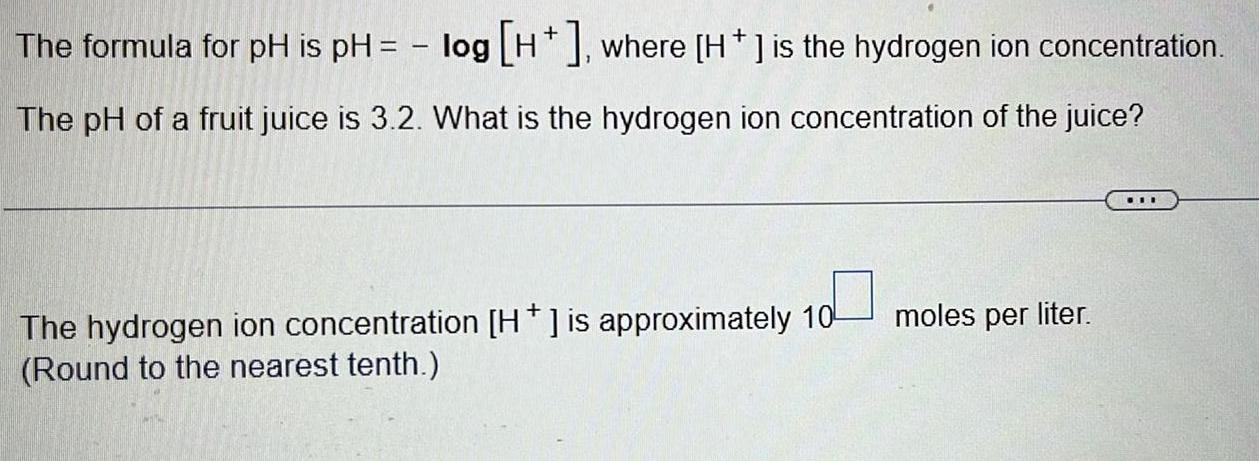Question:

# The formula for pH is pH = -log [H+], where [H + ] is the

Last updated: 7/13/2022The formula for pH is pH = -log [H+], where [H + ] is the hydrogen ion concentration. The pH of a fruit juice is 3.2. What is the hydrogen ion concentration of the juice? The hydrogen ion concentration [H + ] is approximately 10 moles per liter. (Round to the nearest tenth.)